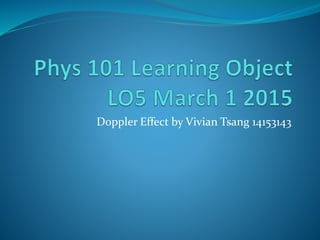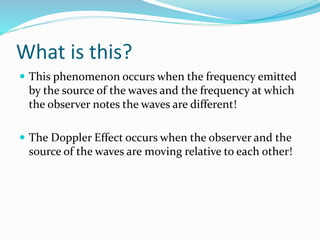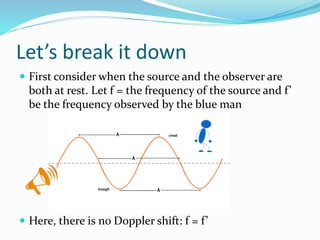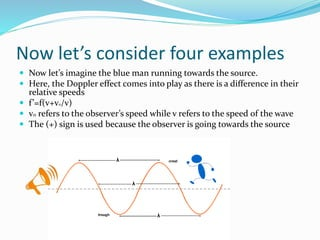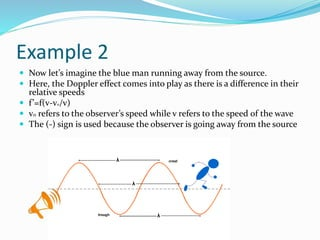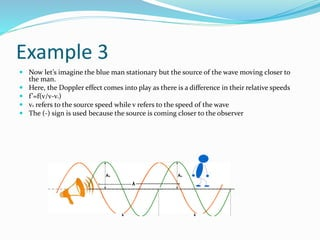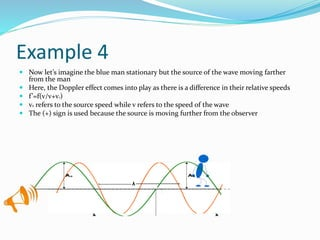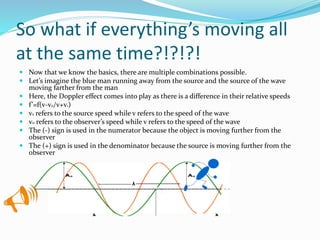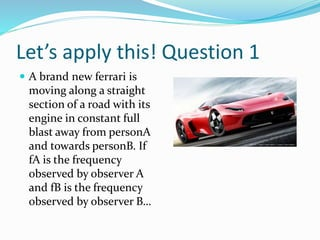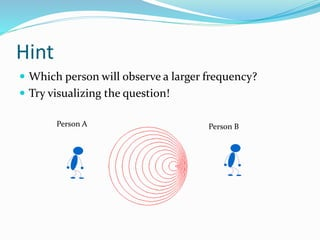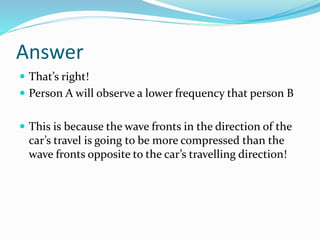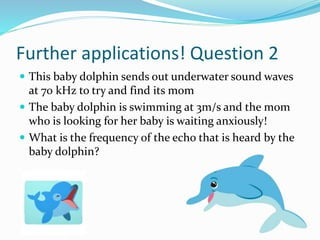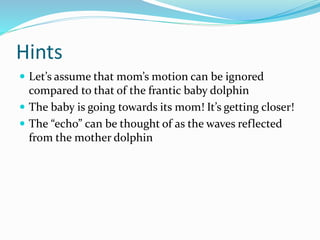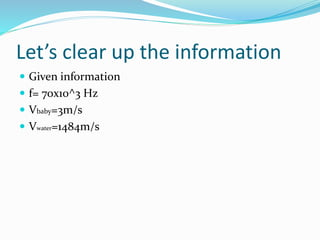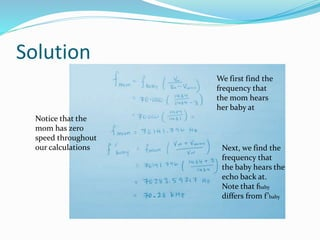1 of 16

### Phys 101 learning object lo5 doppler effect vivian tsang

1. Doppler Effect by Vivian Tsang 14153143
2. What is this?  This phenomenon occurs when the frequency emitted by the source of the waves and the frequency at which the observer notes the waves are different!  The Doppler Effect occurs when the observer and the source of the waves are moving relative to each other!
3. Let’s break it down  First consider when the source and the observer are both at rest. Let f = the frequency of the source and f’ be the frequency observed by the blue man  Here, there is no Doppler shift: f = f’
4. Now let’s consider four examples  Now let’s imagine the blue man running towards the source.  Here, the Doppler effect comes into play as there is a difference in their relative speeds  f’=f(v+vo/v)  v0 refers to the observer’s speed while v refers to the speed of the wave  The (+) sign is used because the observer is going towards the source
5. Example 2  Now let’s imagine the blue man running away from the source.  Here, the Doppler effect comes into play as there is a difference in their relative speeds  f’=f(v-vo/v)  v0 refers to the observer’s speed while v refers to the speed of the wave  The (-) sign is used because the observer is going away from the source
6. Example 3  Now let’s imagine the blue man stationary but the source of the wave moving closer to the man.  Here, the Doppler effect comes into play as there is a difference in their relative speeds  f’=f(v/v-vs)  vs refers to the source speed while v refers to the speed of the wave  The (-) sign is used because the source is coming closer to the observer
7. Example 4  Now let’s imagine the blue man stationary but the source of the wave moving farther from the man  Here, the Doppler effect comes into play as there is a difference in their relative speeds  f’=f(v/v+vs)  vs refers to the source speed while v refers to the speed of the wave  The (+) sign is used because the source is moving further from the observer
8. So what if everything’s moving all at the same time?!?!?!  Now that we know the basics, there are multiple combinations possible.  Let’s imagine the blue man running away from the source and the source of the wave moving farther from the man  Here, the Doppler effect comes into play as there is a difference in their relative speeds  f’=f(v-v0/v+vs)  vs refers to the source speed while v refers to the speed of the wave  v0 refers to the observer’s speed while v refers to the speed of the wave  The (-) sign is used in the numerator because the object is moving further from the observer  The (+) sign is used in the denominator because the source is moving further from the observer
9. Let’s apply this! Question 1  A brand new ferrari is moving along a straight section of a road with its engine in constant full blast away from personA and towards personB. If fA is the frequency observed by observer A and fB is the frequency observed by observer B…
10. Hint  Which person will observe a larger frequency?  Try visualizing the question! Person A Person B
11. Answer  That’s right!  Person A will observe a lower frequency that person B  This is because the wave fronts in the direction of the car’s travel is going to be more compressed than the wave fronts opposite to the car’s travelling direction!
12. Further applications! Question 2  This baby dolphin sends out underwater sound waves at 70 kHz to try and find its mom  The baby dolphin is swimming at 3m/s and the mom who is looking for her baby is waiting anxiously!  What is the frequency of the echo that is heard by the baby dolphin?
13. Hints  Let’s assume that mom’s motion can be ignored compared to that of the frantic baby dolphin  The baby is going towards its mom! It’s getting closer!  The “echo” can be thought of as the waves reflected from the mother dolphin
14. Let’s clear up the information  Given information  f= 70x10^3 Hz  Vbaby=3m/s  Vwater=1484m/s
15. Solution Notice that the mom has zero speed throughout our calculations We first find the frequency that the mom hears her baby at Next, we find the frequency that the baby hears the echo back at. Note that fbaby differs from f’baby
16. Thank you for watching! Thanksfor helping us!